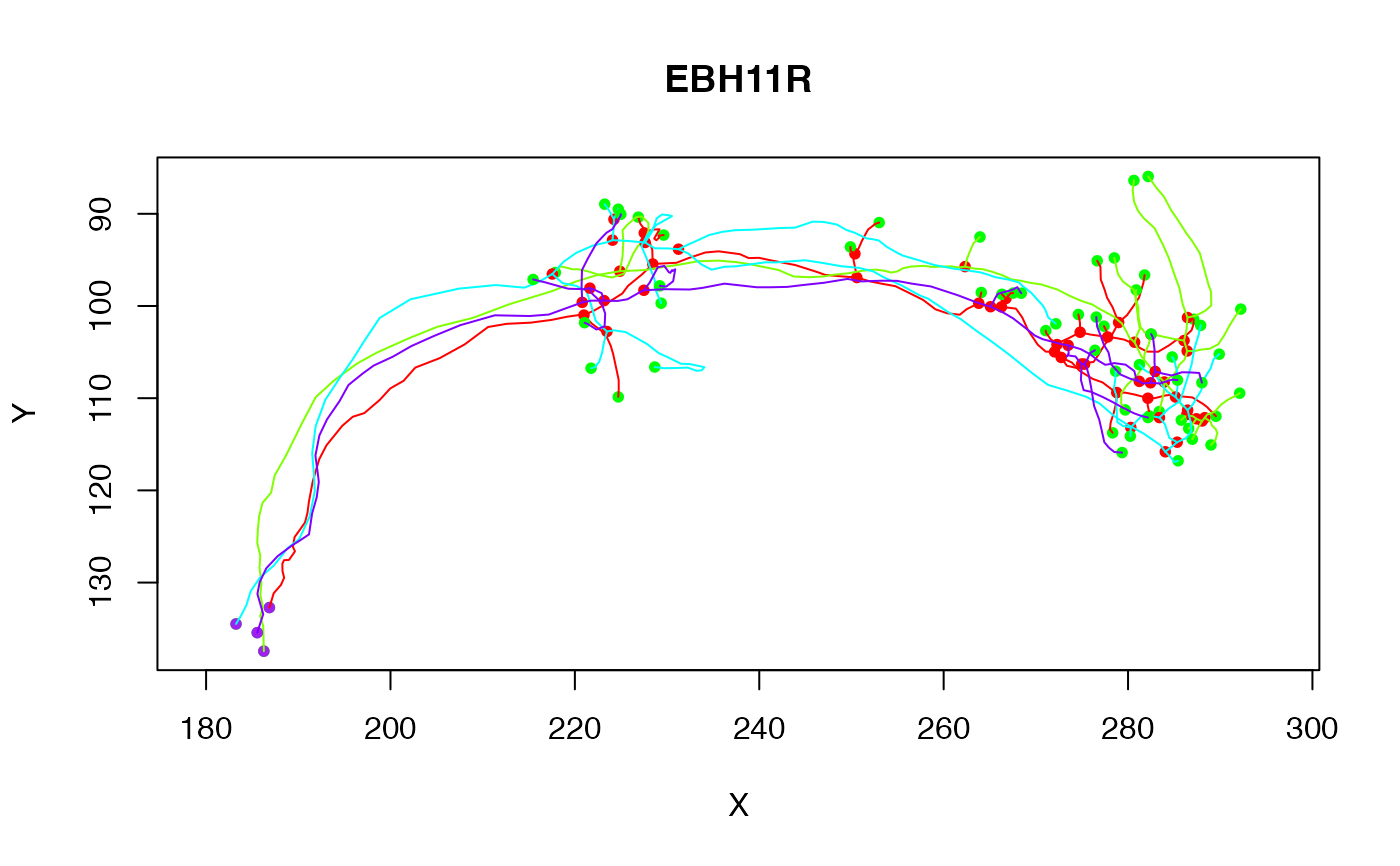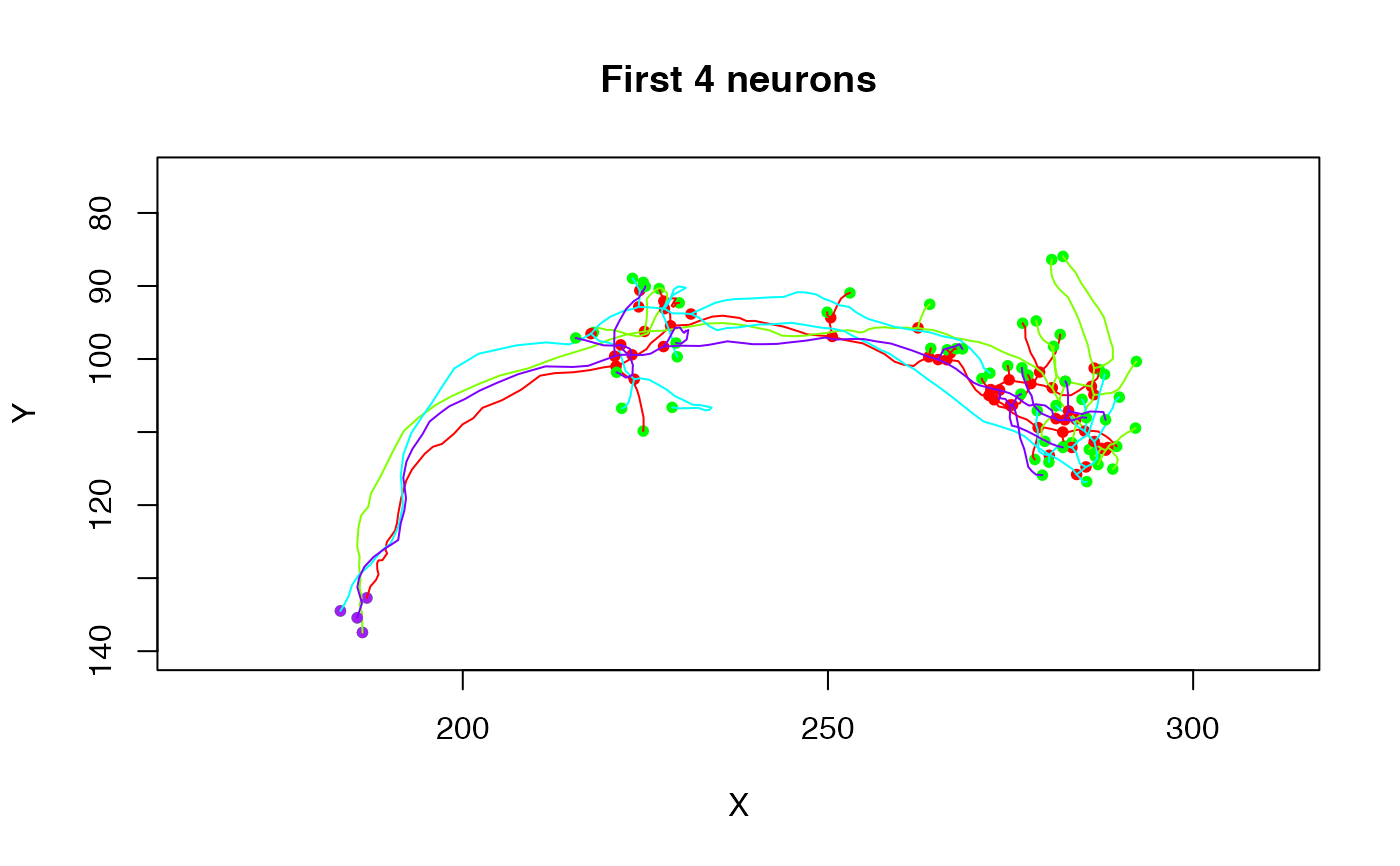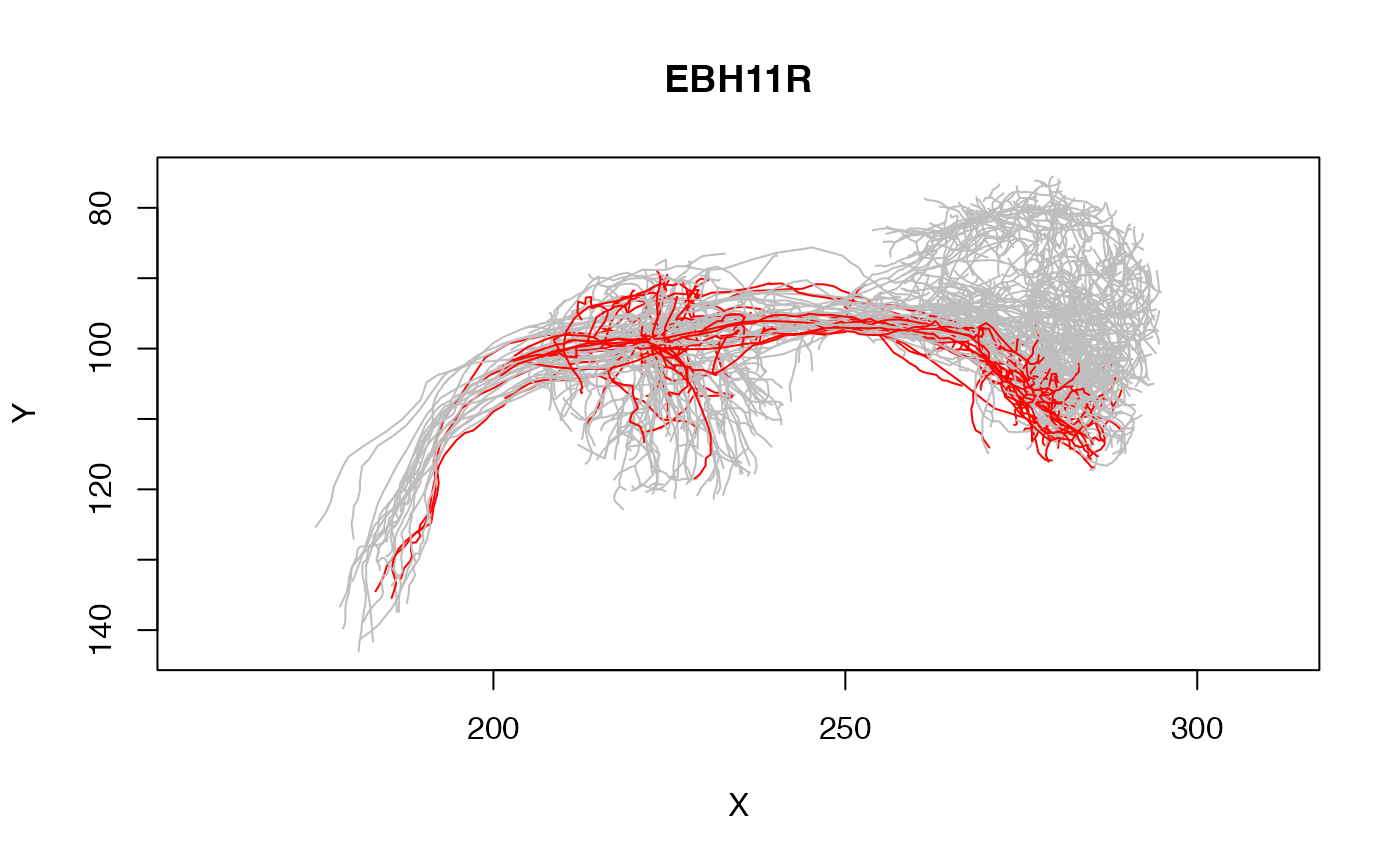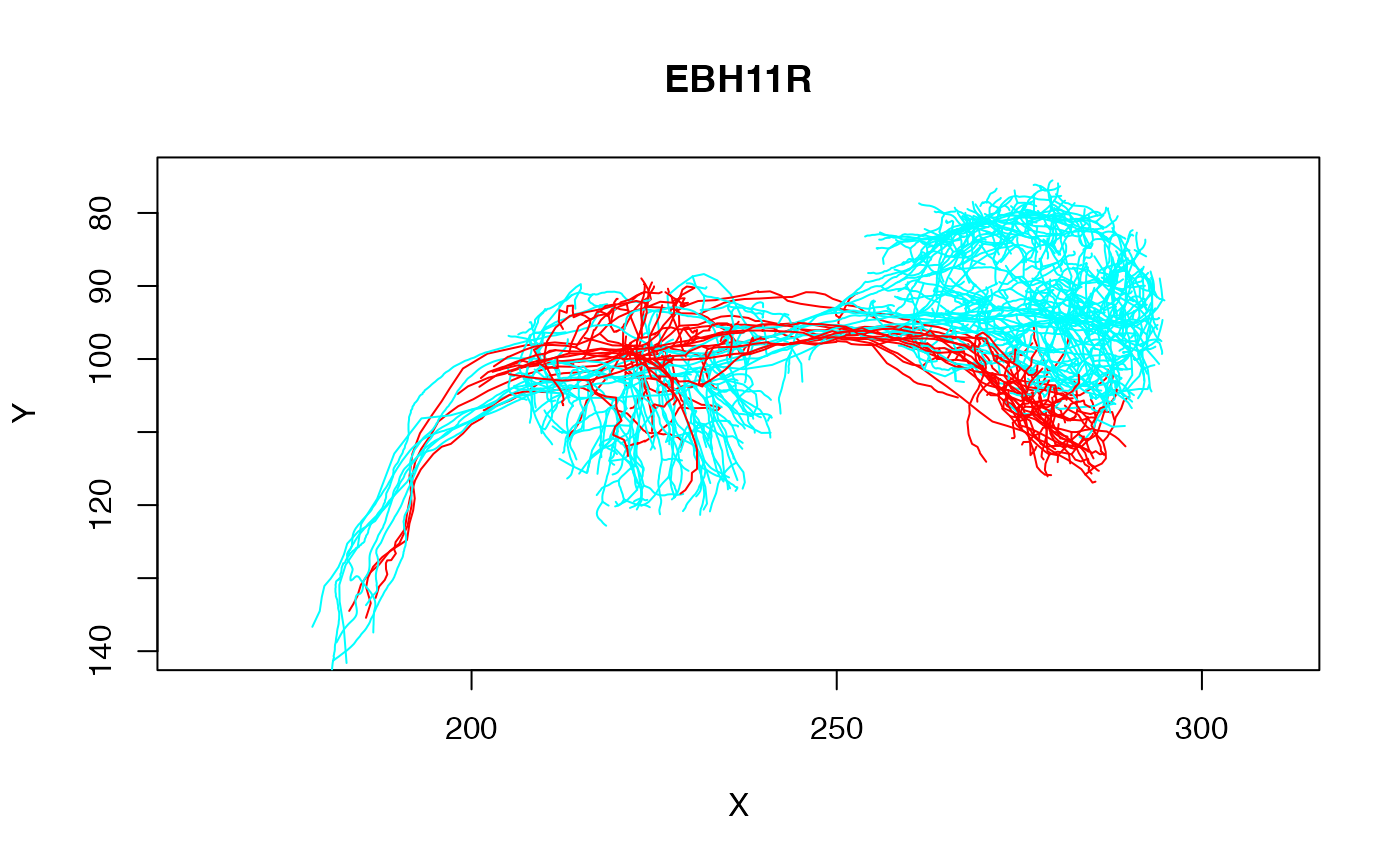2D plots of the elements in a neuronlist, optionally using a subset expression

# S3 method for neuronlist
plot(
x,
subset = NULL,
col = NULL,
colpal = rainbow,
boundingbox = NULL,
soma = FALSE,
...,
SUBSTITUTE = TRUE
)

## Arguments

x

a neuron list or, for plot3d.character, a character vector of neuron names. The default neuronlist used by plot3d.character can be set by using options(nat.default.neuronlist='mylist'). See ?nat for details.

subset

Expression evaluating to logical mask for neurons. See details.

col

An expression specifying a colour evaluated in the context of the dataframe attached to nl (after any subsetting). See details.

colpal

A vector of colours or a function that generates colours

Logical specifying whether to add data to an existing plot or make a new one. The default value of NULL creates a new plot with the first neuron in the neuronlist and then adds the remaining neurons.

boundingbox

A 2 x 3 matrix (ideally of class boundingbox) that enables the plot axis limits to be set without worrying about axis selection or reversal (see details)

soma

Whether to plot a sphere at neuron's origin representing the soma. Either a logical value or a numeric indicating the radius (default FALSE). When soma=TRUE the radius is hard coded to 2.

...

options passed on to plot (such as colours, line width etc)

SUBSTITUTE

Whether to substitute the expressions passed as arguments subset and col. Default: TRUE. For expert use only, when calling from another function.

## Value

list of values of plot with subsetted dataframe as attribute 'df'

## Details

The col and subset parameters are evaluated in the context of the dataframe attribute of the neuronlist. If col evaluates to a factor and colpal is a named vector then colours will be assigned by matching factor levels against the named elements of colpal. If there is one unnamed level, this will be used as catch-all default value (see examples).

If col evaluates to a factor and colpal is a function then it will be used to generate colours with the same number of levels as are used in col.

nat-package, plot3d.neuronlist

## Examples

# plot 4 cells
plot(Cell07PNs[1:4])# modify some default plot arguments
plot(Cell07PNs[1:4], ylim=c(140,75), main='First 4 neurons')# plot one class of neurons in red and all the others in grey
plot(Cell07PNs, col=Glomerulus, colpal=c(DA1='red', 'grey'), WithNodes=FALSE)# subset operation
plot(Cell07PNs, subset=Glomerulus%in%c("DA1", "DP1m"), col=Glomerulus,
ylim=c(140,75), WithNodes=FALSE)